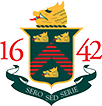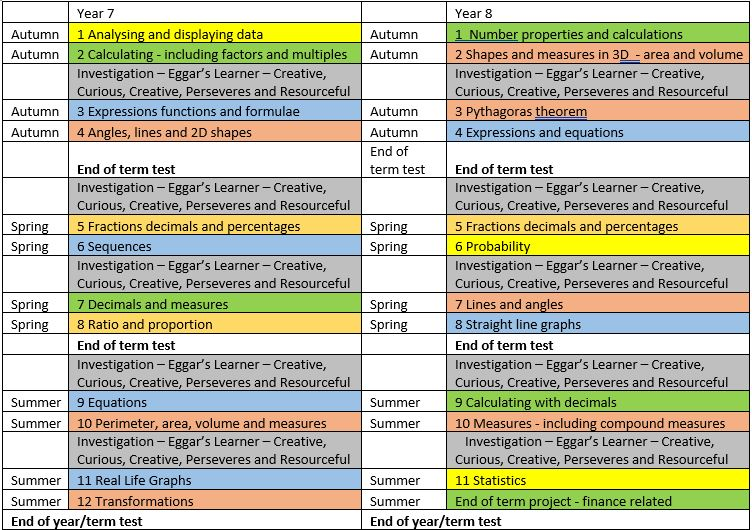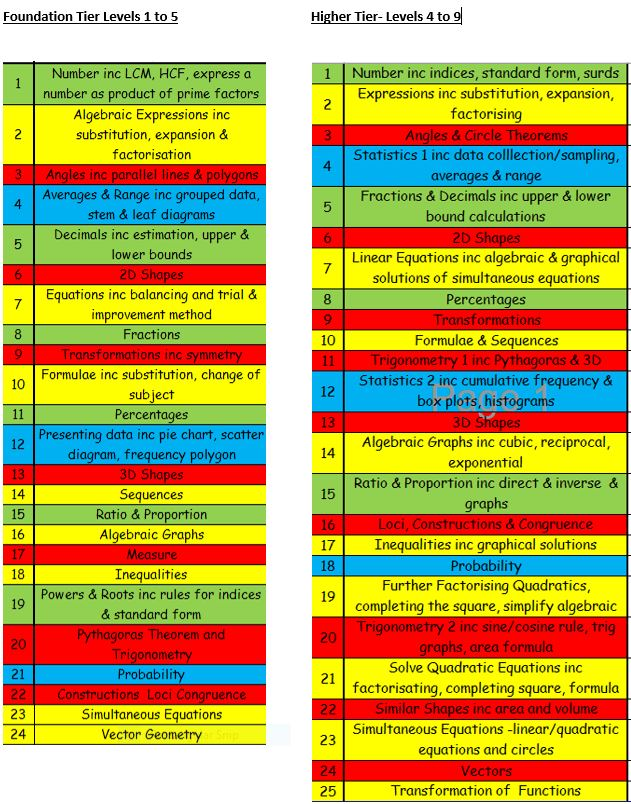#Eggar’s School

01420 541194# Mathematics

### Welcome to the Maths Department  2020 - 2021Mrs J Betteridge

### The Team:

Mrs Kathryn Uffindell               Assistant Curriculum Leader
Mr Aaron Bufton                         Classroom Teacher
Ms Lesley Danks                          Classroom Teacher
Mr Daniel Heaven-Gandy      Classroom teacher
Mrs Linda Hustler                       Classroom Teacher
Mr Luke Whatmough                Classroom Teacher@EggarsMaths

### Introduction to the Department:

In the Mathematics Department, we aim to help students build up their confidence in using mathematical skills and processes and applying them in problem solving.  We endeavour to help make the transition from Primary to Secondary school as smooth as possible, and this year will be using KS2 SATS results and our own baseline line assessment in the first few weeks to set the students.

#### Key Stage 3 Information:

In Key Stage 3, we are constantly developing our curriculum to make it dynamic and relevant to the students we teach.  Our KS3 curriculum has five main strands; Number, Algebra, Ratio and Proportion, Geometry and Statistics. We are also introducing regular half termly investigations which will stimulate, extend and challenge students. These will also encourage students to embrace the different facets of the Eggar’s Learner.

Key mathematical skills are tested regularly while formal assessments take place every term.

Regular reflection on progress and effort is made both in lessons and following informal learning checks and formal assessments.

Homework is set on a weekly basis using a mixture of different topics and four different levels of questions.

The standard of presentation expected of an Eggar’s student in Maths is extremely high

#### Key Stage 3 Overview:#### Assessment in Key Stage 3 Maths

In KS3, students will be formally assessed termly. Their attainment will be measured using bands called “emerging”, “developing”, “secure” and “excelling”. (There will be other smaller assessments during each term).

KS2 results and an initial assessment at the start of Year 7 will determine in which band your son/daughter will start KS3.

Each band has many skills that will need to be developed over KS3. There are five different strands of Mathematics studied, linked to the National Curriculum Programmes of Study for Mathematics at KS3. The five strands are: Number, Algebra, Ratio and Proportion, Geometry and Measures, and Statistics.   The details below show one set of skills within each of four of the strands: Number, Algebra, Geometry and Measures and Statistics.

 YEAR 7 Number:  Four operations and types of number Assessment Criteria Band Students can: Emerging know and use the priority of operations and laws of arithmetic recall multiplication facts up to 10 × 10, multiply and divide by 10, 100, 1000 add, subtract, multiply and divide numbers in different ways using mental and written methods and solve problems using four operations approximate before adding, subtracting, multiplying and dividing use a calculator to solve problems and interpret a calculator display use simple negative numbers recognise and use multiples, factors and prime numbers find common factors and common multiples work out the Highest Common Factor (HCF) and Lowest Common Multiple (LCM) of two numbers round numbers to the nearest 10, 100 and 1000 Developing all the skills in “Emerging” above check answers using estimation and inverse operations solve problems involving time and money using a calculator order positive and negative numbers add and subtract positive and negative numbers and begin to multiply with negative numbers work out the HCF and LCM of two or three numbers recognise and use square numbers, square roots and triangle numbers round decimals to the nearest whole number and to 1 or 2 decimal places Secure all the skills in “Emerging” and “Developing” above recall the first ten square numbers and first 5 cube numbers use mental and written strategies for multiplication, division, addition and subtraction, including problem solving and calculations involving brackets estimate answers to complex calculations add, subtract, multiply and divide positive and negative numbers find all the factor pairs of any whole number carry out calculations involving squares, cubes, square roots and cube roots round to 1, 2 or 3 significant figures Excelling all the skills in “Emerging”, “Developing” and “Secure” above find the HCF and LCM of two or three numbers using Venn diagrams round decimals to any number of decimal places and significant figures YEAR 7 Algebra: Expressions, formulae and functions Assessment Criteria Band Students can: Emerging find outputs and inputs of simple functions. describe simple functions using words or symbols know and use algebraic notation and terminology simplify algebraic expressions by collecting like terms write expressions given a description in words substitute positive integers into expressions and simple formulae written in words and letter symbols write simple formulae using letter symbols identify the unknowns in a formula and a function Developing all the skills in “Emerging” above use arithmetic operations with algebra use brackets with numbers and letters simplify more complicated expressions by collecting like terms write expressions from word descriptions using addition, subtraction and multiplication and to represent function machines substitute into expressions involving powers write and use simple formulae using letter symbols expand expressions involving a single bracket Secure all the skills in “Emerging” and “Developing” above introduce function notation construct expressions using four operations derive formulae from a description expand and simplify expressions involving single brackets factorise an algebraic expression Excelling all the skills in “Emerging”, “Developing” and “Secure” above construct and use expressions using four operations, brackets and powers substitute into formulae and into expressions involving powers derive and use formulae from a description expand expressions involving double brackets factorise an algebraic expression, including variables as factors YEAR 7 Geometry:  Angles Assessment Criteria Band Students can: Emerging identify a right angle as 90 degrees recognise quarter, half and three-quarter turns, parallel and perpendicular lines and acute, obtuse and reflex angles draw and measure acute and obtuse angles using a ruler and protractor label lines and angles estimate the size of angles find missing angles on a straight line and round a point use a ruler and protractor to draw triangles accurately use the rule for the sum of angles in a triangle identify and name types of quadrilaterals Developing all the skills in “Emerging” above identify angle, side and symmetry properties of triangles use the rule for angles on a straight line, angles around a point and vertically opposite angles solve problems involving angles calculate interior and exterior angles and solve angle problems involving triangles use the rule for the sum of angles in a quadrilateral solve angle problems involving quadrilaterals Secure all the skills in “Emerging” and “Developing” above solve complex problems involving angles work out unknown angles involving parallel lines understand how to prove that a result is true use properties of a triangle to work out unknown angles use the properties of isosceles and equilateral triangles to solve problems describe the properties of quadrilaterals, including line and rotational symmetry work out the interior and exterior angles of a polygon Excelling all the skills in “Emerging”, “Developing” and “Secure” above solve complex problems involving angles using geometric rules solve problems using the properties of angles in polygons YEAR 7 Statistics: Analysing data Assessment Criteria Band Students can: Emerging display data and find information from tables, pictograms, bar and bar-line   charts organise data using a tally chart understand, draw and use frequency tables and grouped bar charts calculate averages and range from a set of data compare sets of data using range, mode and median Developing all the skills in “Emerging” above read and construct bar charts (including dual and compound) read and construct charts for grouped data calculate averages and range from a chart or frequency table compare two sets of data using an average and the range Secure all the skills in “Emerging” and “Developing” above identify sources of primary and secondary data and group discrete and continuous data choose a suitable sample size, identify a random sample, and understand how to reduce bias use two-way tables, pie charts, and grouped frequency diagrams draw and interpret scatter graphs, including outliers; describe correlations between two sets of data, and draw and use a line of best fit choose the most appropriate average for a set of data recognise when a graph is misleading Excelling all the skills in “Emerging”, “Developing” and “Secure” above understand stratified sampling find the estimated mean from a set of grouped data recognise when a graph or chart is misleading.
 YEAR 8: Number: Number properties and calculations Assessment Criteria Band Students can: Emerging add and subtract larger numbers and decimals multiply larger numbers and decimals use brackets add, subtract, multiply and divide negative numbers calculate using squares, square roots, cubes and cube roots use index notation for powers of numbers use mental methods to calculate combinations of powers roots and brackets use a calculator to check answers write a number as a product of its prime factors find the Highest Common Factor (HCF) and Lowest Common Multiple of two numbers using Venn diagrams Developing all the skills in “Emerging” above use written methods to add, subtract and multiply with decimals calculate with money estimate answers to calculations use index notation for powers of numbers, including fractional powers estimate the square root of a number use index laws to calculate combinations of powers roots and brackets use prime factor decomposition and Venn diagrams to find the HCF and LCM of 2 numbers Secure all the skills in “Emerging” and “Developing” above work out the laws of indices for positive, negative and fractional powers show that any number to the power of zero is 1 calculate with powers, including fractional and negative powers use and understand the effect of multiplying and dividing by any integer power of 10 use the prefixes associated with powers of 10 Excelling all the skills in “Emerging”, “Developing” and “Secure” above understand how to write a number in standard form and to convert between standard from and ordinary form use prime factor decomposition and Venn diagrams to find the HCF or LCM of two or three numbers YEAR 8 Algebra: Expressions and equations Assessment Criteria Band Students can: Emerging simplify expressions by collecting like terms find outputs and inputs of function machines construct functions from words using algebraic notation solve simple equations and check the solution is correct understand the difference between an expression and an equation, and identify the unknown in an equation use brackets with numbers and variables simplify expressions involving single brackets Developing all the skills in “Emerging” above understand and simplify algebraic powers substitute values into formulae and algebraic expressions involving powers simplify expressions involving brackets, use rules for indices and factorise expressions multiply out double brackets and collect like terms write and simplify algebraic expressions and formulae change the subject of a formula find the inverse of a function solve real life problems using equations solve equations using the balancing method solve equations with the unknown number on both sides Secure all the skills in “Emerging” and “Developing” above understand the meaning of an identity use the index laws in algebraic calculations and expressions write and simplify expressions involving brackets and powers factorise an algebraic expression using numbers and variables as factors substitute integers into expressions construct and solve equations with brackets Excelling all the skills in “Emerging”, “Developing” and “Secure” above understand the meaning of an identity and be able to use reasoning to show an identity write and simplify expressions involving brackets and powers multiply out double and triple brackets and collect like terms factorise an algebraic expression using numbers and variables as factors, including factorising double brackets with positive values YEAR 8 Geometry: Lines, Angles and Construction Assessment Criteria Band Students can: Emerging use a protractor to measure and draw obtuse and reflex angles estimate the size of reflex angles use vertically opposite angles work out the size of unknown angles in a triangle, including isosceles and equilateral triangles accurately draw triangles using a ruler and protractor and pair of compasses accurately draw a net of a 3D shape investigate the sides of a right-angled triangle Developing all the skills in “Emerging” above use alternate, corresponding and co-interior angles to find unknown angles use reasoning to complete mathematical proofs solve geometrical problems using side and angle properties of triangles and quadrilaterals and problems using properties of angles in parallel and intersecting lines find unknown angles by forming and solving equations solve geometrical problems showing reasoning calculate the sum of the interior and exterior angles of a polygon Secure all the skills in “Emerging” and “Developing” above draw accurate diagrams to scale to solve problems construct nets of 3D solids using a ruler and compasses bisect a line and bisect angles using a ruler and compasses construct perpendicular lines using a ruler and compasses draw a locus and use loci to solve problems Excelling all the skills in “Emerging”, “Developing” and “Secure” above draw a locus and use loci to solve problems, including loci of areas YEAR 8 Statistics: Probability Assessment Criteria Band Students can: Emerging use the language of probability and know and use probability notation use a probability scale with words and numbers write probabilities as fractions, decimals and percentages find all the possible outcomes of an event or two simple events use equally likely outcomes to calculate probabilities calculate the probability of an event's not happening use data from an experiment to estimate probabilities collect data from an experiment and make calculations based on results compare and interpret probabilities Developing all the skills in “Emerging” above calculate probability based on equally likely outcomes, compare probabilities calculate probability of A or B happening by counting outcomes calculate the probability of an event’s not happening record data from a simple experiment, estimate probability based on experimental data and make conclusions based on the results use probability to estimate the number of expected wins in a game apply probabilities from experimental data in simple situations Secure all the skills in “Emerging” and “Developing” above identify and find the probabilities of mutually exclusive outcomes and events find the probability of an event's not happening calculate and use the relative frequency of a value to estimate the probability of an event use estimated probability to calculate expected frequencies list all the possible outcomes of one or two events in sample space diagrams or Venn diagrams calculate probabilities of repeated events use tree diagrams to find the probabilities of two or more events Excelling all the skills in “Emerging”, “Developing” and “Secure” above list all the possible outcomes of one or two events in sample space diagrams and Venn diagrams and know the set notation for Venn diagrams calculate probabilities of repeated events, independent and not independent use tree diagrams to find the probabilities of two or more events

In order to move to the next band, a pupil must achieve the criteria for the band they are in and some of the criteria from the next band. A pupil who does not consistently achieve all or most of the required skills could be moved down a band. Hence, as a parent you will be able to check your son/daughter’s progress in Mathematics.

### Key Stage 3 Curriculum Videos by Year Group:

Year 7 Curriculum

### Key Stage 4 Information

At Key Stage 4, students enter one of two pathways, Higher or Foundation, based on their potential and their endeavour throughout Key Stage 3. There is a considerable overlap in the content of these 2 pathways. This allows plenty of time for students to master the skills needed before a final decision on which tier they will sit for the exam in Year 11 has to be made.

Homework is set on a weekly basis, both to consolidate current learning and to revise different topics.

### Assessment in KS4 Maths

In KS4, students will be formally assessed once a term. Their attainment will be measured against the exam board’s GCSE criteria and students will be graded in line with GCSE grade boundaries. (There will be other smaller assessments during each term).

KS3 results and ongoing assessment will determine which tier of assessment band your son/daughter will start on for KS4. This tier may change during KS4 depending on a student’s individual progress.### Examination Boards used:

We use the Edexcel exam board for Mathematics and the AQA Exam Board for Further Mathematics.

GCSE Mathematics

### Other Information

Students from all years take part in the national challenge run annually by the UK Mathematics Trust, and we also send students to local Maths challenges, competing against other schools.